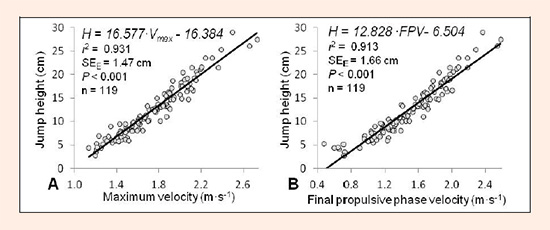Figure 1. Simple linear regression models used to estimate jump height. Model obtained using as the independent variable maximum velocity (A) or final propulsive phase velocity (B). H = jump height (cm); Vmax = maximum velocity; FPV = final propulsive phase velocity; SEE = standard error of the estimate.